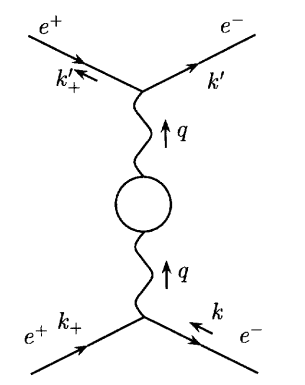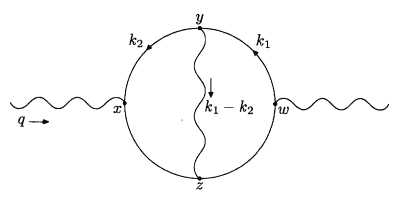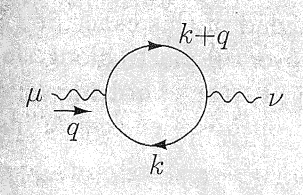# QED Feynmann diagram

## Homework Statement

Write the momentum space integral representation for the following diagram in QED## The Attempt at a Solution

[/B]
##i\mathcal{M}=(-1)\bar{u}(k')v(k'_+)(-ie\gamma^\nu)\left(\frac{-ig_{\nu\sigma}}{q^2+i\epsilon}\right)\left(\frac{-ig_{\mu\rho}}{q^2+i\epsilon}\right)(-ie\gamma^\mu)u(k)\bar{v}(k_+)\displaystyle\int\frac{d^4l}{(2\pi)^4}\times##
##\times tr\left[(-ie\gamma^\sigma)\frac{i(\slashed{l}+\slashed{q}+m)}{(l+q)^2-m^2+i\epsilon}(-ie\gamma^\rho)\frac{i(\slashed{l}+m)}{l^2-m^2+i\epsilon}
\right]##

Is this correct? I'm using the rules on page 801 of Peskin & Schroeder. The main thing I'm not sure about if I should have those ##\epsilon## since I've seen examples where they weren't included.

The ##-1## comes from a closed fermion loop.

Orodruin
Staff Emeritus
Homework Helper
Gold Member
2021 Award
You have two gamma matrices "on the lose". Your amplitude should be a scalar and therefore the gamma matrices must be within a trace or within a spinor bilinear. Basically you need to figure out where to put the gammas corresponding to the top and bottom vertices.

Ah. Well I know the ones I have in the trace should be fine I think and everything would be okay if the other two were inside the trace, but I don't think I want to do that since they're not part of the loop.

Orodruin
Staff Emeritus
Homework Helper
Gold Member
2021 Award
Ah. Well I know the ones I have in the trace should be fine I think and everything would be okay if the other two were inside the trace, but I don't think I want to do that since they're not part of the loop.

Exactly, so where should you have them if not inside the trace?

Another thing I did not notice while on my iPhone: Writing ##u(k)\bar v(k_+)## does not make sense either. Again, the amplitude should be a scalar and ##u(k)\bar v(k_+)## is a matrix.

Well, the slashed terms in the trace are just scalars, so the trace expression reduces to this scalar by the trace of two gamma matricies, which is ##tr(\gamma^\sigma\gamma^\rho)=4g^{\sigma\rho}## and all the indicies contract to leave a scalar expression.

Ah, thanks for that. I'll examine that in a moment.

Orodruin
Staff Emeritus
Homework Helper
Gold Member
2021 Award
Well, the slashed terms in the trace are just scalars

This is not correct. Slashed quantities, i.e., ##p_\mu \gamma^\mu## are still matrices. You cannot put them wherever you want or take them out of the trace without further ado. Anyway, as I said, the trace part is not the problem. The problem is the placement of your ##\gamma^\mu## and ##\gamma^\nu## and the fact that you should not have anything like ##u(k)\bar v(k_+)## in your amplitude.

Edit: Also, what is the time direction in your diagram? Depending on this, you may have to make modifications to which spinors you are using. Regardless of whether you are using time going up or time going right as convention, the lower left particle should be an electron and not a positron based on the direction of the fermion line.

This is not correct. Slashed quantities, i.e., ##p_\mu \gamma^\mu## are still matrices. You cannot put them wherever you want or take them out of the trace without further ado. Anyway, as I said, the trace part is not the problem. The problem is the placement of your ##\gamma^\mu## and ##\gamma^\nu## and the fact that you should not have anything like ##u(k)\bar v(k_+)## in your amplitude.

Edit: Also, what is the time direction in your diagram? Depending on this, you may have to make modifications to which spinors you are using. Regardless of whether you are using time going up or time going right as convention, the lower left particle should be an electron and not a positron based on the direction of the fermion line.

Ahh yes. Sorry, I'm still getting used to the gamma matricies and have so far been used to associating objects with one Lorentz index as being vectors. Anyway, from looking at the Fermion rules in P&S the issue with the ##u(k)\bar{v}(k_+)## ordering should be the spinors in the scattering amplitude being ordered as ##\bar{u}(k')u(k)v(k'_+)\bar{v}(k_+)##. This object is a scalar so I think it should be correct, unless I need to have ##u,v## mixing states.

Now, as for the problem with the gamma matricies. You ask where should I have them if not inside the trace? Well, outside the trace then. This would fit in with P&S saying the terms inside the trace should be a product of fermion propagators with no mention of vertex contributions. But the verticies are on the loop so I think they should contribute to the trace... I was also reading notes earlier that had the gamma matricies in the trace.

With regards to the arrow, I'm pretty sure it's a misprint on the diagram and that the bottom left is a electron and the bottom right is a positron. I will ask my professor.

Orodruin
Staff Emeritus
Homework Helper
Gold Member
2021 Award
Ahh yes. Sorry, I'm still getting used to the gamma matricies and have so far been used to associating objects with one Lorentz index as being vectors. Anyway, from looking at the Fermion rules in P&S the issue with the ##u(k)\bar{v}(k_+)## ordering should be the spinors in the scattering amplitude being ordered as ##\bar{u}(k')u(k)v(k'_+)\bar{v}(k_+)##. This object is a scalar so I think it should be correct, unless I need to have ##u,v## mixing states.
You can view the spinors essentially as 1x4 or 4x1 matrices, respectively. The spinors without a bar are essentially column vectors, while the ones with the bar are row vectors. Thus, an expression like ##\bar u u v \bar v## is essentially (row vector)x(column vector)x(column vector)x(row vector) = (1x1 matrix)x(4x4 matrix) and therefore is a matrix. The way of constructing the amplitude correctly is to do the following for each open fermion line:
1. Start from the end of the line (i.e., go against the arrow) and write down the spinor (with a bar) corresponding to that external line. If it is an electron coming out of the interaction, this will be a ##\bar u##, if it is a positron going into the interaction, it will be a ##\bar v##.
2. Follow the line in the arrow starting from the first vertex you encounter: Write down alternatingly the vertex factors (including the gamma matrix that appears) and the propagators. End when you reach the last vertex.
3. Write down the spinor that you encounter at the end of step 2. This will be a ##u## for an incoming electron and a ##v## for an outgoing positron.
Note that you cannot take the gamma matrices out of this construction for each fermion line. The scalars you can take out, but the gamma matrix is a matrix that acts on the spinors.

From what you've said I would expect the part in front of the integral and the trace then to be

##\bar{u}(k')(-ie\gamma^\mu)v(k'_+)\left(\frac{-ig_{\mu\rho}}{q^2+i\epsilon}\right)\left(\frac{-ig_{\mu\rho}}{q^2+i\epsilon}\right)\bar{v}(k_+)(-ie\gamma^\nu)u(k)##

I have my doubts about this being correct since the metric tensor is still there. I'd imagine this needs to go between the spinors.

Orodruin
Staff Emeritus
Homework Helper
Gold Member
2021 Award
The metric tensor is fine anywhere, it is simply a number (as you have written it in component form) and will have the appropriate contraction with the gamma matrices, i.e., you can just as well use it to lower some indices. The part that is not correct in that expression is that both of your metric tensors have the same indices - this part you had right in your original post.

Ah, sorry, that was a typo. So the correct answer for the amplitude then would be

##i\mathcal{M}=(-1)\bar{u}(k')(-ie\gamma^\mu)v(k'_+)\left(\frac{-ig_{\mu\rho}}{q^2+i\epsilon}\right)\left(\frac{-ig_{\sigma\nu}}{q^2+i\epsilon}\right)\bar{v}(k_+)(-ie\gamma^\nu)u(k)\displaystyle\int\frac{d^4l}{(2\pi)^4}\times##
##\times tr\left[(-ie\gamma^\rho)\frac{i(\slashed{l}+\slashed{q}+m)}{(l+q)^2-m^2+i\epsilon}(-ie\gamma^\sigma)\frac{i(\slashed{l}+m)}{l^2-m^2+i\epsilon}
\right]##

or

##i\mathcal{M}=(-1)\bar{u}(k')(-ie\gamma_\rho)v(k'_+)\left(\frac{-i}{q^2+i\epsilon}\right)\left(\frac{-i}{q^2+i\epsilon}\right)\bar{v}(k_+)(-ie\gamma_\sigma)u(k)\displaystyle\int\frac{d^4l}{(2\pi)^4}\times##
##\times tr\left[(-ie\gamma^\rho)\frac{i(\slashed{l}+\slashed{q}+m)}{(l+q)^2-m^2+i\epsilon}(-ie\gamma^\sigma)\frac{i(\slashed{l}+m)}{l^2-m^2+i\epsilon}
\right]##

Is this correct? I can obviously simplify further by bringing the factors of ##i## and ##e## etc but I'm not worried about that.

Also, is it correct to have the ##i\epsilon## in the denominator of the propagators? The rules in P&S have the ##i\epsilon## but for example, when P&S examines Compton Scattering there are no ##i\epsilon## terms in the propagator, so I'm not sure when they should or shouldn't be included.

Sorry for the messy propagators by the way. Physics Forums doesn't seem to like Feynmann slashes.

Orodruin
Staff Emeritus
Homework Helper
Gold Member
2021 Award
Also, is it correct to have the i\epsilon in the denominator of the propagators? The rules in P&S have the i\epsilon but for example, when P&S examines Compton Scattering there are no i\epsilon terms in the propagator, so I'm not sure when they should or shouldn't be included.

Yes. The epsilons are simply pole prescriptions and should technically be there. They tell you which poles to pick up in the propagators. The limit of epsilon going to zero is implied.
Sorry for the messy propagators by the way. Physics Forums doesn't seem to like Feynmann slashes.
Feynman slashes are not part of any LaTeX distribution as far as I am aware. You always have to import something to do it properly, so it is no surprise it does not work here.

Thank you very much for the help! Much appreciated!

Figured there was no point creating a new thread.

Anyway, I want to evaluate the following diagram in momentum space.Using the Feynmann rules and what I've learned from the previous problem I would expect to get

##i\mathcal{M}=\epsilon^*_\mu(q)\epsilon_\nu(q)(-1)\displaystyle\int\frac{d^4k_1}{(2\pi)^4}\int\frac{d^4k_1}{(2\pi)^4}\frac{-ig_{\rho\sigma}}{(k_1-k_2)^2+i\epsilon}\times##
##tr\left[(-ie\gamma^\mu)\frac{i(\slashed{k_1}+m)}{k_1^2-m^2+i\epsilon}(-ie\gamma^\rho)\frac{i(\slashed{k_2}+m)}{k_2^2-m^2+i\epsilon}(-ie\gamma^\sigma)\frac{i(\slashed{k_2}+\slashed{q}+m)}{(k_2+q)^2m^2+i\epsilon}(-ie\gamma^\nu)\frac{i(\slashed{k_1}+\slashed{q}+m)}{(k_1+q)^2m^2+i\epsilon}\right]##

Is this correct? I'm mostly worried about the photon propagator and if it should be in the loop.

Also, on page 245 of Peskin and Schroeder they give the following diagramand evaluate it as

##(-ie)^2(-1)\displaystyle \int\frac{d^4k}{(2\pi)^4}tr\left[\gamma^\mu\frac{i}{\slashed{k}-m}\gamma^\nu\frac{i}{\slashed{k}+\slashed{q}-m}\right]##

Why is there no mention of the initial and final states ##\epsilon_\mu(q)## and ##\epsilon^*_\nu(q)##? More importantly, why are they using those odd propagators when they state that the loop is an electron loop?

Orodruin
Staff Emeritus
Homework Helper
Gold Member
2021 Award
Why is there no mention of the initial and final states ##\epsilon_\mu(q)## and ##\epsilon^*_\nu(q)##? More importantly, why are they using those odd propagators when they state that the loop is an electron loop?

First question: This is the correction to the photon propagator, if you would evaluate it in a real process it would be attached to either an internal line or an out state.

Second question: These are the normal fermion propagators, simply written in a different form. Note that:
$$\frac{i}{k-m} = i\frac{k+m}{(k+m)(k-m)} = i\frac{k+m}{k^2-m^2}.$$
(I am not writing the slashes as it doesn't appear correctly anyway).

Ahh right okay. But how would the ##i\epsilon## terms be included then? I'm guessing since they're infinitesimal we can just put them in?

Is my amplitude for the first diagram in my last post correct?